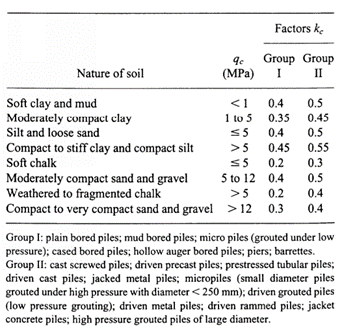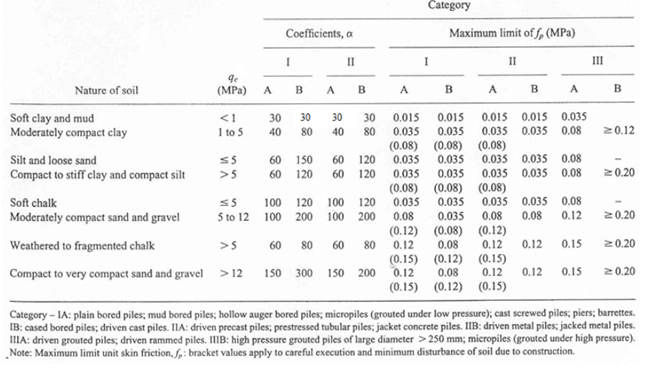top of page

# LCPC CPT method for pile axial capacity in ALLCPT program

ALLCPT program provides a pile capacity analysis tool which can be used to calculate pile axial capacity for various pile types based on the imported CPT data. The pile axial capacity calculation is based on the LCPC CPT method proposed by Bustamante and Gianeselli (1982). The users can select Tool >> Pile Axial Capacity Tool from the top menu to open the pile axial capacity tool, which is shown in the figure below.Figure 1 - Open Pile Axial Capacity Tool

For pile axial capacity, the ultimate unit end bearing resistance fb is calculated from the equivalent average cone resistance qca which is multiplied by an end bearing coefficient Kc according to the following relationship:The end bearing coefficient Kc can be estimated in accordance with the table below.Figure 2 - End bearing resistance coefficient Kc

The equivalent average cone resistance qca is calculated based on the figure below.Figure 3 - Equivalent average cone tip resistance calculation

In ALLCPT program, the equivalent average cone resistance qca is calculated in the following steps:

• Step 1: calculate the average tip resistance qca', mean at the tip of the pile by averaging qc values over a length ranging from a=1.5D below the pile tip to a=1.5D above the pile toe (D is the pile diameter).

• Step 2: Remove qc values in the zone which are higher than 1.3 times the mean of the cone tip resistance qca', mean and those are lower than 0.7 times the mean of the cone tip resistance qca', mean as shown in figure.

• Step 3: Calculate the equivalent average cone tip resistance qca by averaging the remaining cone tip resistance qc values over the same zone that were not removed.

The ultimate unit side friction fs is calculated by measured qc values divided by a friction coefficient alpha-LCPC in according to the following relationship:The friction coefficient alpha-LCPC can be determined by the table below:Figure 4 - Skin friction coefficient alpha-LCPC

The pile axial capacity tool in ALLCPT program is shown in the figure below. The supported pile section types are circular section, rectangular section, octagonal section, H section, Pipe Section and user-defined section.Figure 5 - Pile axial capacity tool in ALLCPT

There are three different design approaches provided in the pile axial capacity tool and summarised as below:

• Limit state design - AS 2159-2009

• Limit state design - AASHTOFigure 6 - Design approaches for pile axial capacity

The following figure shows the results available for graphical plot for the design approach of "Working load design". This selection dialog can be opened with double-clicking mouse on any sub-figure. The graphical plot on that sub figure will be then updated with the selected plotting type once this selection dialog is closed.Figure 7 - Axial capacity analysis results for the design approach of working load design

The following figure shows the results available for graphical plot for the design approach of "Limit state design - AS 2159-2009".Figure 8 - Axial capacity analysis results for Limit state design - AS 2159

The following figure shows the results available for graphical plot for the design approach of "Limit state design - AASHTO".Figure 9 - Axial capacity analysis results for Limit state design - AASHTO

In addition to the pile axial capacity results, pile settlement under axial load is calculated based on Fleming’s approach (Fleming 1992) in ALLCPT program. The settlement results can be viewed by the users by clicking the “Pile settlement” button on the toolbar of the pile capacity analysis tool. The input parameters relevant to the settlement analysis can be input by the users through “Pile Capacity Analysis Option” dialog as shown in Figure 6 under the section of “Pile Settlement Analysis Option”. A typical pile settlement analysis result dialog is shown in the figure below.Figure 10 - Pile settlement analysis results from ALLCPT program

733 views